##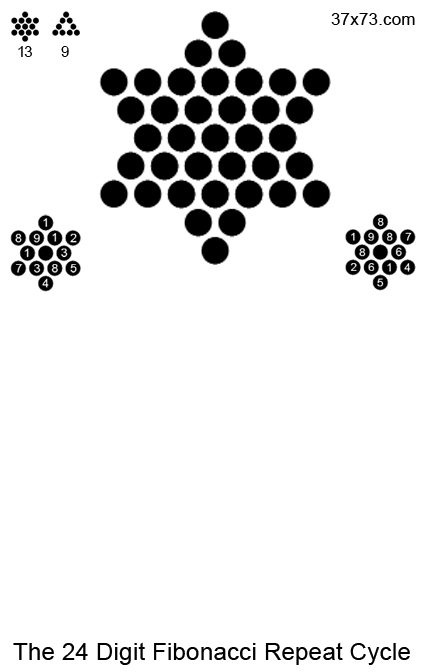Theologians have long known that the mind of God is infinite and surpasses all human understanding. This sobering fact suggests that any codes left by the Divinity would be far too sophisticated and complex for human beings to ever hope to comprehend. The great wonder of the Three Seven Code is that it can be understood!

A code like this can have many meanings depending on the context. Theologically the 3 clearly represents the Trinity and the 7 days of creation or the 7 archangels. Scientifically, the primary meaning of the THREE SEVEN CODE is the GOLDEN RATIO also known, as the Divine Proportion. The Golden Ratio lies at the crossroads of science and religion. Based in the Fibonacci Series the Golden Ratio is so universal in its application throughout the sciences it has become its own 'Meme' or running joke, simply because it is found almost everywhere in the natural world.

## 12³ The New Jerusalem, Jesus Christ and the Holy Spirit

The name Jesus Christ actually hides the 12th cube in its geometry. The 12th cube (1728 or 12 x 12 x 12) are the dimensions of the
New Jerusalem, described in the book of Revelation. By looking at the sum 2368 as a portion or gnomen of a cube of cubes
(64 x 64 or 4096) we find that the remaining invisible portion comes to the 12th cube, i.e. 4096 - 2368 = 1728.

Is this a cornerstone?

What is really curious about this configuration and this miniature magnetic model in particular, is that the first person I showed it to, declared it looked just like a cornerstone.  Gnomen's such as these are a standard part of geometry. So geometrically this is a corner whose sum is equivalent to the name Jesus Christ-2368.

A similar gnomen can be made of the Seventh word of the Bible, the last word of the first verse, 'The Earth' —Ha-aretz.

296
2 x 2 x 2 x 296 = 2368

This is a double-gnomen or the difference between the 8th and the 6th cube (512 - 216 = 296). The whole cube here is an analog of the name Jesus in Greek, since the whole cube is 8 x 8 x 8, i.e., Jesus-888. Eight of these 296-gnomens produce another cube of Jesus Christ-2368.

The Jesus Christ-gnomen made of 37 cubes of 64,  is also part of a much larger cube.

16³

16 x 16 x 16 = 4096

This number and shape is the visual approximation of the Golden Ratio, 1.6 To some sticklers this approximation may not seem accurate enough to qualify. Sadly, this notion often occurs in those who lack an understanding of the real practice of mathematics in the sciences. The fact that the irrational numbers pi, phi and e have infinite decimal expansions is intellectually fascinating but practically pointless. For thousands of years engineers got by with an approximation of pi based on the simple fraction of twenty-two over seven:

22/7 = 3.1428...

The ancient approximation of pi

Scientists today still use approximations in their calculations and it is well established that they need only but the first few decimals for the work to be done with necessary accuracy. This is all to say that 1.6 or 16 is a 2-digit perfect approximation of the Golden Ratio. Visually the human eye cannot tell the difference between 1.6 and 1.618, they are practically indistinguishable. This cube of 16 can also be perfectly divided according to the Golden Ratio.

4096/1.6 = 2560

2560 x 1.6 = 4096

Another fascinating component of this arrangement is how the 12th cube relates to the name Jesus Christ. When we divide 1728 in the 2368, we get a repeating decimal that is an approximation of the Fine Structure Constant (see Psalm 137). If we take the cube of the New Jerusalem as a metaphor for the physical universe, then Jesus Christ-2368 is the vital spirit of that universe in the form of the Fine Structure Constant.

12³ = 1728

2368/ 1728 = 1.370370370....

Similarly, should we take the name 'The Holy Spirit' in Greek, and multiply it by the proportion of the Golden Ratio we find the cube of the New Jerusalem:

The Holy Spirit
το Πνευμα Aγιον = 1080

1080 x 1.6 = 12³

The basic geometric component of this construction is (27) the ordinal sum of Light Aur in Hebrew and the number books in the New Testament. The temple like configuration is composed of 40 of these cubes, which come together to produce a larger cube based on the dimensions of the New Jerusalem described in the book of Revelation.

The Greek name for Jerusalem has a very similar geometry based again on the 3rd and 12th cubes.

Jerusalem (Ιερουσαλημ) = 864

One of the marvels of the Golden Ratio is how its proportionality both ascends and descends—phi only transcendental number that does this. One over phi, is an inverse proportion but with phi it still retains its same proportionality. Here again the number 1080 (The Holy Spirit) provides the perfect proportion using the biblical approximates: 1/φ = 0.6 and φ = 1.6

The New Jerusalem - (The Holy Spirit x 1/φ)

1080 x 0.6 = 648
1728 - 648 = 1080

Should we increase the accuracy of our approximation of phi we find a double twelve-fold truth, where the twelve-fold dimensions are encoded both the name of the Lord (Kυριος-89 ordinal) and the Tree of Life (החיים-עץ 233).

1728 x 0.618 = 1068
1728 x 1.618 = 2796

12 x 89 = 1068
12 x 233 = 2796

To my knowledge, 12-cubed is the only cube that can incorporate Fibonacci numbers in ratio to its dimensions.

φ = 1.6180

1/φ = 0.6180

Check the FAQs!

Scientists know that numbers, and prime numbers in particular, are the universal language. With this in mind, should an alien species attempt contact with the earth they would likely use some sort of prime number reference, indeed this was the idea behind Carl Sagan's book and movie 'Contact.'

If aliens and scientists are so smart wouldn't God be even smarter?

We know from the mounting evidence of theistic mathematics that God is the master mathematician. His mathematics are at the same time both incredibly complex and ingeniously simple. It is this repeated simplicity in solution that is the hallmark of the master mathematician. With this in mind we take the two prime numbers 3 and 7 to the very core of mathematics itself, to the very building blocks of math. In this way we can see that the 3 and 7 are a very specific, scientific reference.

In mathematics, a series of prime numbers can be a reference to another number. This is called the Fundamental Theorem of Arithmetic. It is called the 'Fundamental Theorem' because when it comes to numbers there is nothing more basic than prime factorization.  In this way the prime numbers 3 and 7 become a clear reference to the number 21, a number of incalculable importance to theistic mathematics.

The number 21 is also a reference to the Greek letter phi (
φ) and phi is nothing less than the mathematical symbol of the Golden Ratio.

Prime Factors of 21 = 3 x 7
21st Greek Letter = φ

φ = The Golden Ratio = 3, 7

φ = 1.61800339...

What the continual repetition of the number pattern of 3 and 7 is truly saying to us is this: I AM Phi, I AM the Golden Ratio, I AM the Divine Proportion. And it is saying it over and over and over again, forever. In the book of Exodus God tells Moses that His name is I AM.

אהיה = I AM = 21
אהיה = I AM = φ

אהיה = I AM = The Divine Proportion

There is another cryptic reference to the Three Seven Code in the book of the Revelation of Saint John. The reference clearly refers to Christ, but the secret of it lies in the Greek spelling of the name of David. Here the Greek from the Textus Receptus displays just what this 'Key' may be, in that the Greek spelling of David, gives us a sum of 21 or 3 x 7 the fundamental key of the Three Seven Code. Please note the Chapter and Verse.

Revelation 3:7
Chapter THREE Verse SEVEN

"He that hath the key of David"
ο εχων την κλειδα του Δαβιδ

David = Δαβιδ = 3 x 7

There is a great deal of evidence that the primary code of the Bible is pointing directly at the Golden Ratio. Some of the most compelling are the instructions God gives to build His sacred objects. Noah's ark, the altar of sacrifice and the Ark of the Covenant are all designed to the Divine Proportion. God's most sacred name 'Yahweh' features aspects related to phi. Even the colors of the curtains in the tabernacle accord to the Golden Ratio.

These correlations were made using the Phibar program (available from the Goldennumber website) and shows just one permutation (out of hundreds) of how the colors of the Bible coordinate according to the Golden Ratio.

## RECENT HISTORY

There is another way that we know that the meaning of the Three Seven Code is the Golden Ratio. For the last 20 years a small group of amateur and professional mathematicians have been searching for Three-Seven relationships in the numbers of the Bible. In this obscure field, the number 373 is universally acknowledged as a primary component of the eventually named Three Seven Code. The number 373 refers to the Logos of the Gospel of John.

In the beginning was the Word, and the Word was with God, and the Word was God.

The word for 'WORD' here, in the Greek, is LOGOS λόγος.  John 1:1 is one of most famous and influential verses in the entire Bible. It has had a profound influence on Christian Theology. The word logos itself, has had enormous global influence in the history of the world and in particularly the history of science. The following words all end in the very specific suffix,-Ology, which comes from the Greek word Logos.

Every -Ology

Logic = Logos

Clearly, this is an important word to the very history of man and science itself.

But how does it relate to the Golden Ratio?

Euclid, (the father of geometry) in his famous book on mathematics, the Elements, first defined the Golden Ratio. He used a specific word, a word that would later be echoed through the work of the likes of Justin Martyr, Augustine and the Apostle John. The word Euclid uses for the Golden Ratio is Logos.

Ratio = Logos
Logos = 373

Quite literally, the Apostle John was referencing the Logos of Euclid, the Divine Proportion. To the Jews of that day, the word meant God. Many have thought it to mean the same. It can mean many things, but in the light of all the THREE's and SEVEN's of the Bible, it means in a very real way the Golden Ratio.

To see just how influential, the Golden Ratio is in our world and history itself, go to the best website on the subject, that thoroughly explains in it full detail. Or simply run a google image search on the Golden Ratio + 'Anything'.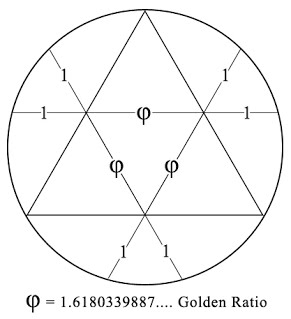One of the standard methods of determining phi (the Golden Ratio) through geometry, is through the use of an equilateral triangle with a circle in circumference, as seen above. Here, when the lines that bisect the triangle have a length of one, the area of the line that transverses the triangle, will be in the Divine Proportion. This division of the first verse into these sections has been known for some time now. Now, however, we can see that it is a confirmation of the Golden Code of the Bible.

John 1:1
"'In the beginning was the Logos, and the Logos was with God and the Logos was God.'
The only ratio that is simultaneously one and with one is the golden section."

-Scott Olsen, The Golden Section

## THE GOLDEN NAME

The very name of Jesus Christ, the most important name of the Bible and the most contentious name in history, accords itself to the Golden Ratio and its inverse. The graphic below displays this ideal ratio in the numbers of Greek gematria.These three all important numbers are in a 3:5:8 proportion, all of which are Fibonacci numbers. Curiously and all too appropriately the number 358 is the sum of the Hebrew word for 'Messiah.'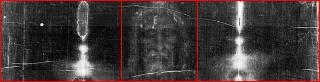This Shroud of Turin portraiture is based on the Divine Proportion

We see this pattern again when we employ the Greek ordinal for 'The Name of the Lord Jesus Christ.'

The Name of the Lord Jesus Christ

Το Ονομα Κυριου Ιησου Χριστου = 370

370 x φ(1.6) = 592

1480 - 888 = 592

When we add the number of the name of Christ to the sum of the first verse we get, at very long odds, the 19th Fibonacci number.

Genesis 1:1 = 2701 Christ = 1480

2701 + 1480 = 4181

4181 = 19th Fibonacci Number

These facts display a correspondence with Jesus Christ and Golden Ratio. Incredibly, we see the same type of reference in the very words that Euclid uses to mathematically define the Divine Proportion. In the Elements, Euclid describes the Golden Ratio as:

The Extreme and Mean Ratio = ακρος και μεσος λογος

Looking at this simple phrase in ancient Greek, we find a double reference to the name of Jesus in both Hebrew and Greek.

Extreme (ακρος) = 391 = Jesus (Hebrew)

Mean Ratio (μεσος λογος) = 888 = Jesus (Greek)

The Golden Spiral, one of the most common manifestations of the Golden Ratio, can be formulated by a series of squares that continually form the Golden Rectangle. When we follow this same pattern, now using 37 as the base number, we create a Golden Rectangle and Spiral that produces the name of Jesus Christ.

The above GIF displays how the prime number 37 and the name Jesus Christ in Greek are positively correlated with the golden spiral. What's missing is the 925 square in the lower left hand corner.

925 = JESUS CHRIST
(English)

Increasingly we are finding more and more evidence of strong correlations with the English translation with the name Jesus with the mathematics of the Bible and in particular the mathematics of the first verse. Read:

There is a 60 digit repeat cycle (see below) that is found in the terminal digits of Fibonacci numbers. If we look at just the first three triplets, we see a rather obvious pattern, to those with even an elementary understanding of Biblical gematria.

1,1,2,3,5,8,3,1,4...

(112) (358) (314)

In Hebrew, these three are of the strongest theology:

112 = Lord God

358 = Messiah

314 = Almighty

Should we look at the Fibonacci numbers themselves, a similar pattern emerges, starting at 21 (φ) with the God’s given name ‘I AM that I AM,’ all ordinal.

1,1,2,3,5,8,13,21,34,55,89,144...

I AM  אהיה = 21

Spirit  רוח  = 34

Lord God  אדני יהוה  = 55

Lord (Kυριος) = 89

Lord Jesus Christ  אדני יהושע המשיח = 144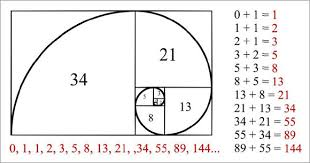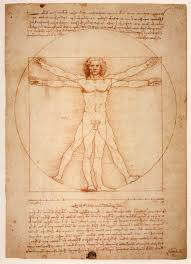## WHEELS WITHIN WHEELS

In the book of Ezekiel (Eze.1:16), the prophet has a vision and describes seeing 'Wheels within Wheels.' This passage has produced many colorful ideas about just what Ezekiel saw, including many dubious ideas such as UFO's.

However, in a world that did not yet have a modern scientific understanding of a logarithmic spiral or even an Archimedean spiral (first understood by the book On Spirals (225BC) by Archimedes) an ancient human would be at a lost for words to describe such a perplexing shape as the spiral.

Considering that the codes of the Bible, again and again, point us to the Golden Ratio and the Divine Proportion, a Golden Spiral is an exact fit for what Ezekiel described as 'Wheels within a Wheels.' This is an apt description of continuing progressive circularity.

WHEELS WITHIN WHEELS

The squaring of the circle is an ancient mathematical challenge that confounded some of the greatest minds in history. It was ultimately proven to be impossible using a finite number of steps. However, we see that when we employ the name Jesus Christ in its Biblical Hebrew and Greek forms, they correlate in two significant ways, the second being a very precise approximation of pi.

The name Jesus Christ in Hebrew and in Greek unite the square and circle in compelling ways

## SCIENTIFIC EVIDENCE

The Fibonacci Sequence is one of the most important number series in all of science. It is absolutely correlated with the Golden Ratio, in that with every new Fibonacci number, the ratio between them comes closer and closer to the irrational number of Phi (1.6180339...) the Divine Proportion.

One of the lesser known facts about the Fibonacci series is that there a 60-digit cycle that repeats infinitely in the final digit of each of the Fibonacci number.

The Black Numbers = Repeat Cycle
` 0 1 1 2 3 5 8 13 21 34 55 89 144 233 377`
The 60 Digit Repeat Cycle
0,1,1,2,3,5,8,3,1,4,5,9,4,3,7,0,7,7,4,1,5,6,1,7,8,5,3,8,1,9,0,
9,9,8,7,5,2,7,9,6,5,1,6,7,3,0,3,3,6,9,5,4,9,3,2,5,7,2,9,1

The Fibonacci series infinitely approximates with ever increasing accuracy the Divine Proportion or Phi (1.6180339...).

3/2 = 1.5, 5/3 = 1.666..., 8/5 = 1.6, 13 / 8 = 1.625, 21/13 = 1.61, 34/21 = 1.619, 55/34 = 1.617, 89/55 = 1.618, etc. etc. on into infinity...

Since the Fibonacci series has an infinite repeat cycle 60 digits its worth examining the first 60 decimal digits of Phi (1.6180339...).

1.618033988749894848204586834365638117720309179805762862135448

Sum Calculator
To check the math copy and paste the numbers and commas into the sum calculator in the above link.
6,1,8,0,3,3,9,8,8,7,4,9,8,9,4,8,4,8,2,0,4,5,8,6,8,3,4,3,6,5,6,3,8,1,1,7,7,2,0,3,0,9,1,7,9,8,0,5,7,6,2,8,6,2,1,3,5,4,4,8  = 294

Lord Jesus Christ = 294

This number, 294 is also the sum of the phrase in Greek of the Key of David.

The Key of David (η κλεις Δαβιδ) = 294

And the 'Key of David' is David times David or David squared.

21 = Δαβιδ = David
14
דוד = David

21 x 14 = 294

When you place these numbers on a circle they reveal the universally known pattern of a clock. Here the hours of the day correspond to either five or zero; the sum of all twelve being a Biblical 40. The 12 numbers of the clock-face subdivide the hour into 5 minute units.

40

The Bible highlights the number 40 in many ways. This number is intimately related to the Golden Ratio and the Fibonacci which perfect the Golden Ratio. 40 days and nights (the most common 40 of the Bible) creates a Golden Ratio of one day squared: (40 days = 24 x 40 = 960 hours// 960 x 1/φ (0.6) = 576 = 24^2).

The Fibonacci Sequence has an infinite number of terminal repeat cycles. The first is 60 (seen below) the second is a 2 digit cycle of 300 digits long, followed by a 1,500 digit cycle, 15,000 and 150,000, ad infinitum. The sum of every repeat cycle is a multiple of 40. Moreover, even the subsets of these cycles and the subsets of the subsets of these cycles are multiples of 40.

Fibonacci Triple-Digit Cycle, 1-1500  = 748,000 = 40 x 18,700

1-300  = 134,800 = 40 x 3370
301-600  = 156,800 = 40 x 3920
601-900  = 155,800 = 40 x 3895
901-1200  = 152,800 = 40 x 3820
1201-1500  = 147,800 = 40 x 3695

Another feature of the Fibonacci Series is the fact that every 19th Fibonacci is a multiple of 37. This is important, in that the codes of science and the Bible both can be solved, typically, with a Star of David in a configuration of 37 units. The hexagon of this Star will always be always 19.

●   ●

●   ●   ●   ●   ●   ●   ●

●   ●   ●   ●   ●   ●

●   ●   ●   ●   ●

●   ●   ●   ●   ●   ●

●   ●   ●   ●   ●   ●   ●

●   ●

The red dots number Nineteen

The series of every 19th Fibonacci also has a 60 digit repeat cycle. This pattern is found by only counting the terminating digit of every 19th Fibonacci number. This repeat cycle has the exact same digits of the former, only in the exact opposite order. This again, is the Mirror Logic of the first verse.

Every 19th Fibonacci = Multiple of 37

Standard 60 Digit Repeat Cycle
0,1,1,2,3,5,8,3,1,4,5,9,4,3,7,0,7,7,4,1,5,6,1,7,8,5,3,8,1,9,0,9,9,8,7,5,2,7,9,6,5,1,6,7,3,0,3,3,6,9,5,4,9,3,2,5,7,2,9,1

Multiples of 37 Repeat Cycle
1,9,2,7,5,2,3,9,4,5,9,6,3,3,0,3,7,6,1,5,6,9,7,2,5,7,8,9,9,0,9,1,8,3,5,8,7,1,6,5,1,4,7,7,0,7,3,4,9,5,4,1,3,8,5,3,2,1,1,0

From this evidence we can see that there is a strong corroboration in the Fibonacci Series (i.e. the Golden Ratio) and the number 37 seen in the first verse, a number that is a primary component of the Three Seven Code. The fact that it is exactly the opposite of the standard 60-digit and not offset in the 60 different ways that it could be, is a clear indication of design.

The rings of Saturn and many other of its features correspond to the Divine Proportion

Every 37 = Every 73

Another feature of the Fibonacci Series shows an an even stronger correlation to the first verse. The Protoverse (Genesis 1:1) displays in its prime factors a very unique design, which is the very seed and icon of the Three Seven Code. We see this code in the greatest of all patterns in the universe: the Fibonacci Series and the Golden Ratio. Here the 3 & 7 and the 7 & 3 of Genesis 1:1 come together again in a fundamental and infinite way.

Every 37th Fibonacci = Multiple of 73

Genesis 1:1 = 37 x 73

`37th Fibonacci = 24,157,817 = 73 x 330,929`
`74th Fibonacci = 1,304,969,544,928,657 = 73 x 17,876,295,136,009`
`111th Fibonacci = 70,492,524,767,089,125,814,114 = 73 x 965,651,024,206,700,353,618`
`On and on to Infinity`

## THE GOLDEN CLOCK

Every hour that you have ever lived and every clock that you have ever seen has been a witness to the Three Seven Code that indicates the Golden Ratio and the Divine Proportion. Indeed, every minute that you have ever lived and are currently living, testifies to God's Divine Proportion. At the 37th minute of every hour, and 37th second of every minute, since the beginning of time the Golden Ratio has chimed a secret beat two separate ways.

When you apply the Golden Ratio to a circle, it is called the Golden Angle. Its measure of degrees is 137, the same as the Fine Structure Constant of the entire universe.

The Golden Ratio (Modern Greek Ordinal) = 137

Golden Angle = 137
Fine Structure Constant = 137

Multiplying the reciprocal of phi (the Golden Ratio), i.e. the inverse proportion, by the number of minutes in a hour, we find the Three Seven Code hiding in plain sight for all eternity.

60 x 0.618 = 37.0

The Golden Ratio once again hits 37 minutes when we multiple phi (1.618) by the number of minutes in an hour.

60 x 1.618 = 97

97 = 1 hour and 37 minutes

97 = The Golden Ratio (Hebrew)

Immediately after the 97th decimal place of phi is the first appearance of the number sequence 37. It also coincides with the first appearance of the number 137, the most important number in physics. All the digits up to the first appearance of the number 137, the Fine-structure Constant and the Golden Angle, equals 441 or 21 squared.

1.6180339887498948482045868343656381177203091798057
62862135448622705260462818902449707207204189391137
Following the number 391 (Jesus in Hebrew) is the Golden Angle itself.

Amazingly, the sum of the numbers up to this most important number of science is precisely 441, Hebrew for Truth Amet, and the square of 21, 'I AM', Eheyeh.

6,1,8,0,3,3,9,8,8,7,4,9,8,9,4,8,4,8,2,0,4,5,8,6,8,3,4,3,6,5,6,3,8,1,1,7,7,2,0,3,0,9,1,7,9,8,0,5,7,6,2,8,
6,2,1,3,5,4,4,8,6,2,2,7,0,5,2,6,0,4,6,2,8,1,8,9,0,2,4,4,9,7,0,7,2,0,7,2,0,4,1,8,9,3,9,1, = 441

Sum Calculator
To check the math copy and paste the numbers and commas into the sum calculator in the above link.

21² = 441

(3 x 7 x 7 x 3)

The number 137 is perhaps the most mysterious and famous number in all of science, especially physics. Some of the greatest minds of the 20th century have pondered at the enigma of 137 and sought after its mystery like it was the holy grail.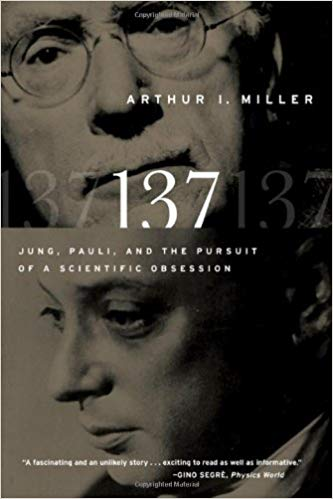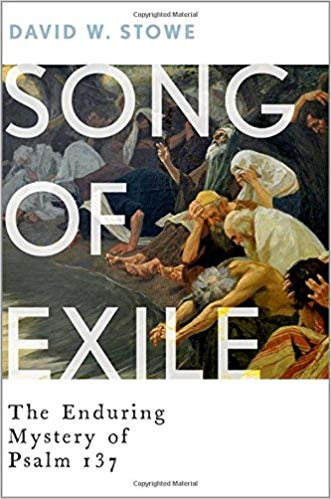Arthur I. Miller's and David W. Stowe's books both struggle with the question of 137.

SPOILER ALERT: You don't need to read all about ancient alchemy or have a Ph.D in physics to understand the underlying importance of the 137. It is quite simply, another crystal clear reference to the Golden Ratio (the Golden Angle = 137) which is penultimate meaning of the Three's and Seven's, which is the very signature of the Lord Jesus Christ, the Most High God, creator of heaven and earth.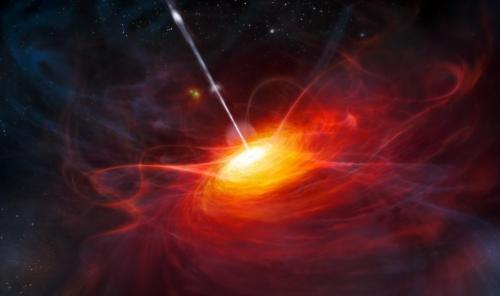## The Lord's Prayer

Below you will see The Lord's Prayer KJV. Employing only the letters without spaces (this is typical of many other known configurations of polymorphic grammatica) you can see that the prayer from Matthew 6:9 has precisely 256 letters, which as a power of two is already a potent mathematical entity.

As 256 is also 16^2, the prayer should be tiled as seen below.

O   U   R   F   A   T   H   E   R   W   H   I   C   H   A   R

T   I   N   H   E   A   V   E   N   H   A   L   L   O   W   E

D   B   E   T   H   Y   N   A   M   E   T   H   Y   K   I   N

G   D   O   M   C   O   M   E   T   H   Y   W   I   L   L   B

E   D   O   N   E   I   N   E   A   R   T   H   A   S   I   T

I   S   I   N   H   E   A   V   E   N   G   I   V   E   U   S

T   H   I   S   D   A   Y   O   U   R   D   A   I   L   Y   B

R   E   A   D   A   N   D   F   O   R   G   I   V   E   U   S

O   U   R   D   E   B   T   S   A   S   W   E   F   O   R   G

I   V   E   O   U   R   D   E   B   T   O   R   S   A   N   D

L   E   A   D   U   S   N   O   T   I   N   T   O   T   E   M

P   T   A   T   I   O   N   B   U   T   D   E   L   I   V   E

R   U   S   F   R   O   M   E   V   I   L   F   O   R   T   H

I   N   E   I   S   T   H   E   K   I   N   G   D   O   M   A

N   D   T   H   E   P   O   W   E   R   A   N   D   T   H   E

G   L   O   R   Y   F   O   R   E   V   E   R   A   M   E   N

The Lord's Prayer = 3003 (o)

3,003 = 77th Triangle (T77)

This triangle (T77) can appropriately be called the Christ Triangle or the triangle of the trinity of Christ, since, 77 = Christ.

C  =  3

H  =  8

R = 18

I  =  9

S = 19

T = 20

= 77

The number 16 is another important coding system. Its reference is phi, the Golden Ratio. Gary Meisner has a wonderful website dedicated to it called the Goldennumber.net.

God uses 1.6 as a Biblical approximate for phi, for two reasons: It is to the human eye, visually indistinguishable from more accurate representations of phi. Second, at the time of the composition of the original text, numbering systems and mathematics as a whole did not include decimal expansions. One of the great moments in the history of mathematics was the discovery of irrational numbers, a discovery that postdates the composition of the Tanakh.

The ordinal sum of these English letters is 3003. This is another important number in theistic mathematics because it is the sum of the first 8 words of the Bible.

In the beginning God created the heavens and the earth and the earth...

בראשית ברא אלהים את השמים ואת הארץ והארץ

302         296         407         395         401         86         203         913

= 3003

The ellipsis at the end of the sentence, represent what these 8 words mean. The first 7 words of the Bible indicate the first verse as well as anticipating the 7 days of creation. The inclusion of the 8 words indicates the beginning of the rest of the Bible.

The number 8 in the context of Scripture means the eternal return. Like a musical octave, there are 7 whole notes and the 8th note makes the octave. We see this in the Passion Week of Christ. On Friday, the 6th day, He is crucified. On Saturday, the day of rest and the 7th day, He lays in the tomb. On the 8th day, the new day of the new week He is resurrected.

888 = Jesus

3003
77
3773

Genesis 1:1 = 37 x 73

##SHARE THIS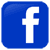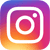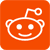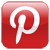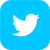"Nothing prevents us, and the momentum of our knowledge requires it…
to interrelate the order of the universe and the God of religion. For the believer,
God stands at the beginning of their speeches; for the physicist, at the end of them."

— Max Planck

Subpages (2):